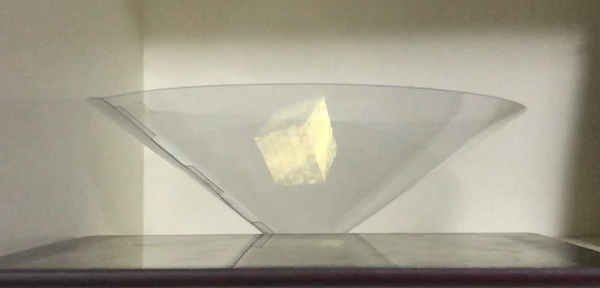# 裸眼3D小实验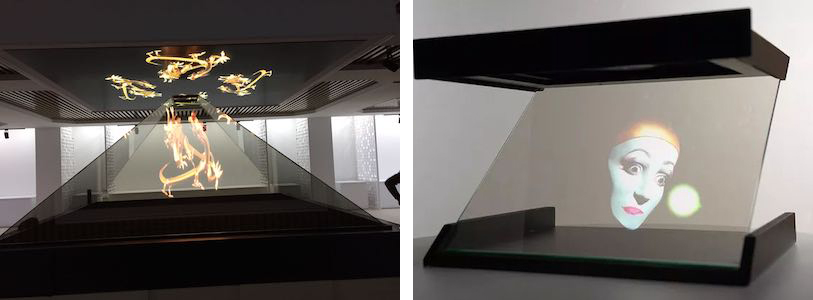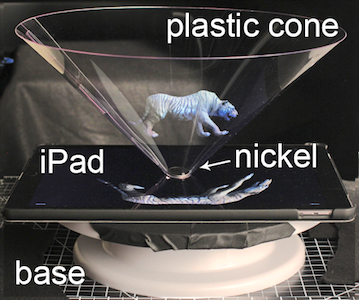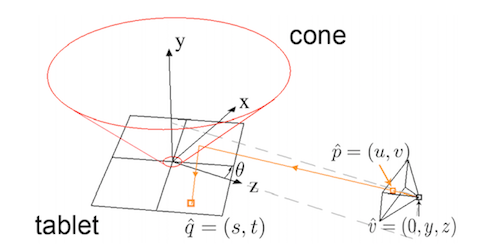Kinect和iPhoneX的人脸ID都用到了结构光技术，其主要用于3D重建，获取物体表面形状信息。原理不难理解，向物体表面投射已知结构的光，然后拍摄图像，根据图像上结构的形变来计算物体表面(深度)信息，在这个实验里，我用的比较简单的格雷码结构光。

### 结构光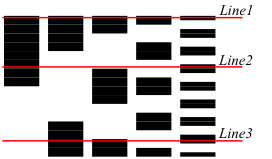``````encodePattern(idx) {
for (y = 0 : height - 1) {
for (x = 0 : width -1) {
int gray = binaryToGray(x);              // 坐标x转为格雷码
bool one = (gray >> idx) & 1;            // 第idx位是否是1
image[x, y].color = one ? white : black; // 像素点颜色
}
}
}``````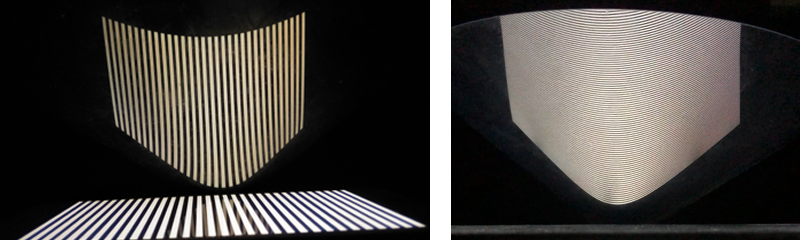``````decodePattern(images) {
for (idx = 0 : images.size) {
for (y = 0 : height - 1) {
for (x = 0 : width -1) {
bool one = isPixelWhite(images[idx][x, y]); // 判断该像素点编码为0还是1
if (one) {
pattern[x, y] |= 1 << idx;               // 第idx位赋1
}
}
}
}

for (y = 0 : height - 1) {
for (x = 0 : width -1) {
pattern[x, y] = grayToBinary(pattern);          // 格雷码转二进制
}
}
}``````

``````uchar *p = image[x, y];
p = patternX >> 8;       // r
p = patternX & 0xFF;     // g
p = patternY >> 8;       // b
p = patternY & 0xFF;     // a``````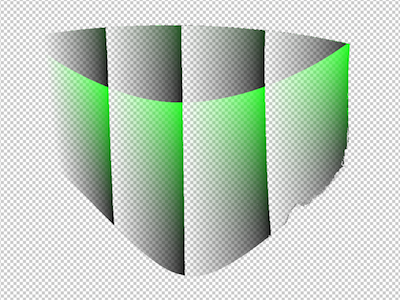### Post Process

``````_frameBuffer = FrameBuffer::create("PostProcess", FRAMEBUFFER_WIDTH, FRAMEBUFFER_HEIGHT);
DepthStencilTarget* dst = DepthStencilTarget::create("PostProcess", DepthStencilTarget::DEPTH_STENCIL, FRAMEBUFFER_WIDTH, FRAMEBUFFER_HEIGHT);
_frameBuffer->setDepthStencilTarget(dst);``````

``````Material* material = Material::create(materialPath);
Texture::Sampler* sampler = Texture::Sampler::create(srcBuffer->getRenderTarget()->getTexture());
material->getParameter("u_texture")->setValue(sampler);

Mesh* mesh = Mesh::createQuadFullscreen();

``````Rectangle defaultViewport = getViewport();

// draw into the framebuffer
setViewport(Rectangle(FRAMEBUFFER_WIDTH, FRAMEBUFFER_HEIGHT));
FrameBuffer* previousFrameBuffer = _frameBuffer->bind();

// main scene
_scene->draw();

// post process
setViewport(defaultViewport);
previousFrameBuffer->bind();

clear(CLEAR_COLOR, Vector4(0, 0, 0, 1), 1.0f, 0);

``````#ifdef OPENGL_ES
precision highp float;
#endif

// Uniforms
uniform sampler2D u_texture;
uniform sampler2D u_mapTexture;

// Inputs
varying vec2 v_texCoord;

void main()
{
vec4 map = texture2D(u_mapTexture, v_texCoord);
vec2 mapUV = vec2((map.b * 256.0 + map.g) / 4.0, (map.r * 256.0 + map.a) / 4.0);
gl_FragColor = texture2D(u_texture, mapUV);
}``````

u_texture是3D场景正常渲染的画面，u_mapTexture是结构光解码后的像素映射图，mapUV的计算则是将rgba解压为x、y坐标(u、v)，如下左右分别是形变前和形变后的图像。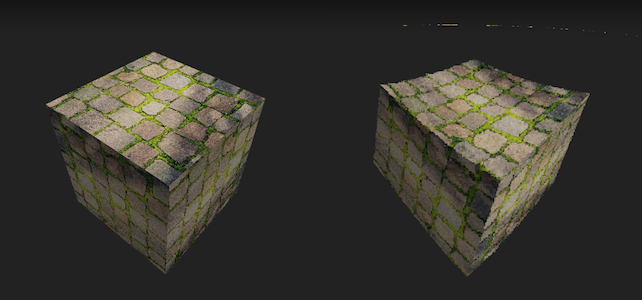### 实验结果